# NCERT Solutions for Class 10 Maths Chapter 15- Probability

NCERT Solutions for Class 10 Maths Chapter 15- Probability

Probability is an area of mathematics that deals with numerical descriptions of what will happen in the future, or whether something is true or not. This is a high-scoring but challenging Chapter in Class 10 Maths. As a result, you must be conversant with the tips and tactics required to quickly handle numerical issues. In this regard, we provide detailed NCERT Solutions for Class 10 Maths Probability, which include many types of sums that may be encountered in exams.

NCERT solutions for Class 10 Maths Chapter 15- Probability | Free PDF Download

NCERT Solutions for CBSE Class 10 Mathematics Chapter 15 Probability will help you to improve your math board exam score. Use our expert solutions to figure out how many coloured balls are in a bag or to prove that a certain event will be impossible. Learn how to apply the probability formula to come up with answers based on the number of favorable outcomes and the total number of outcomes.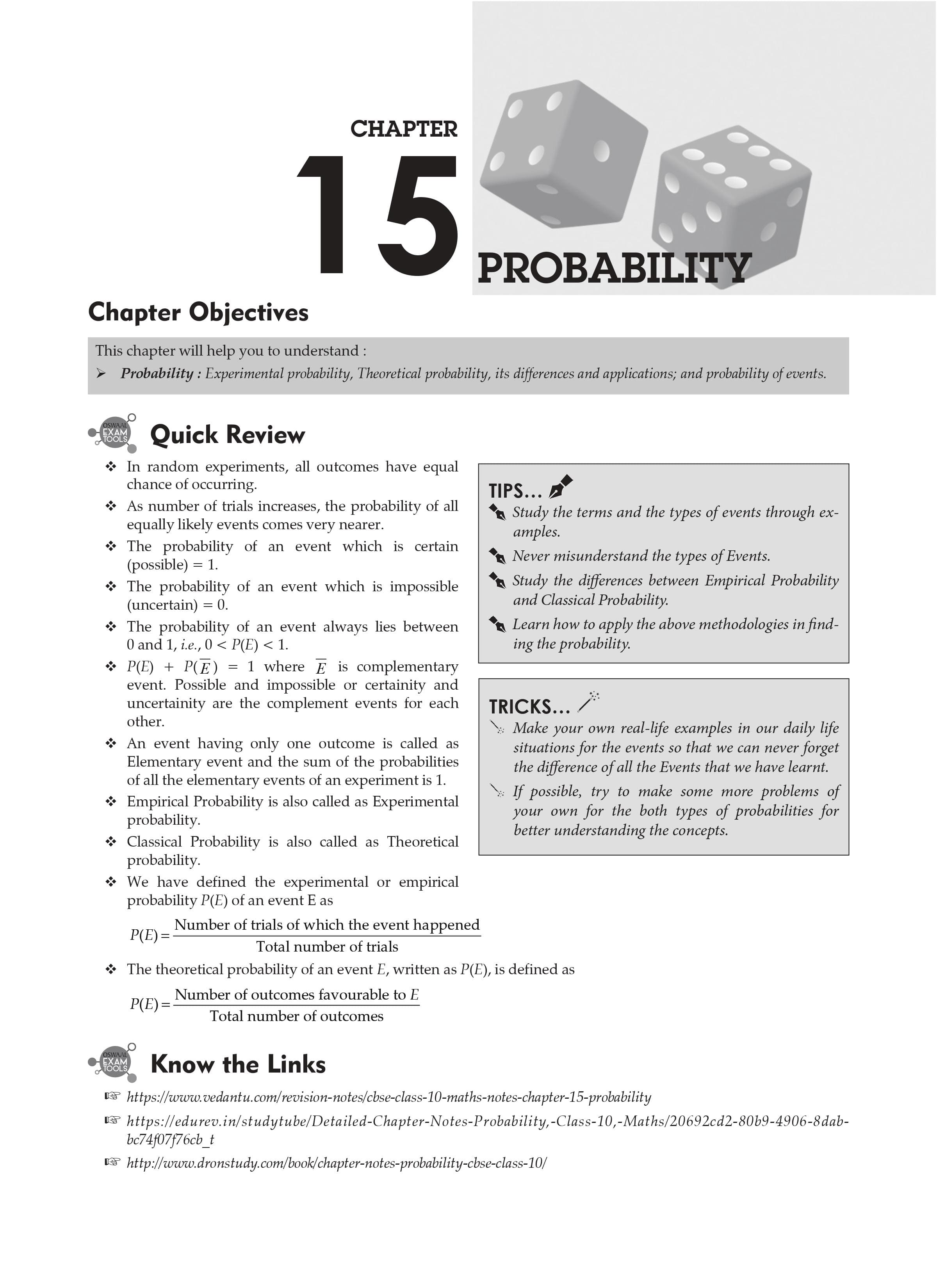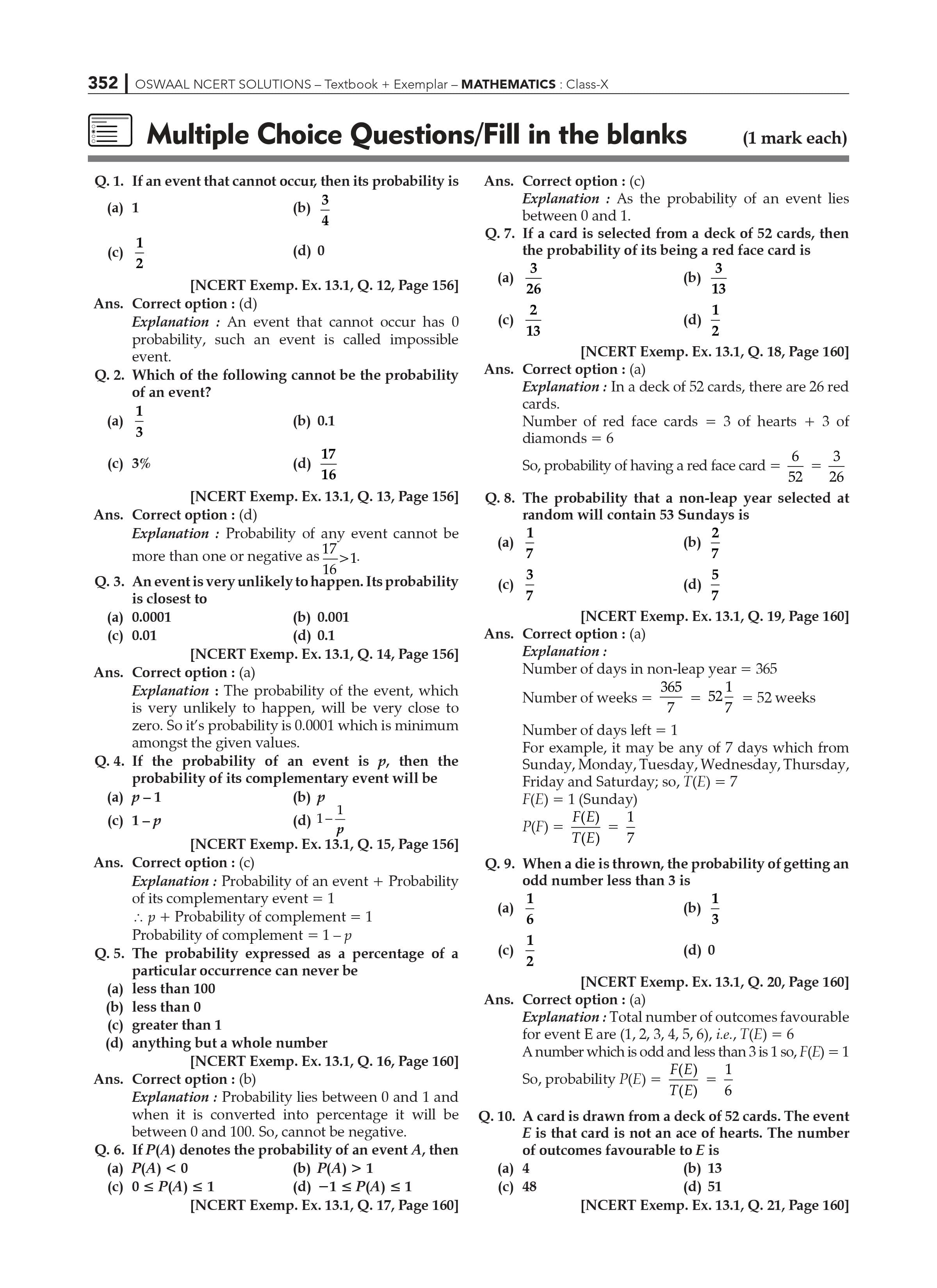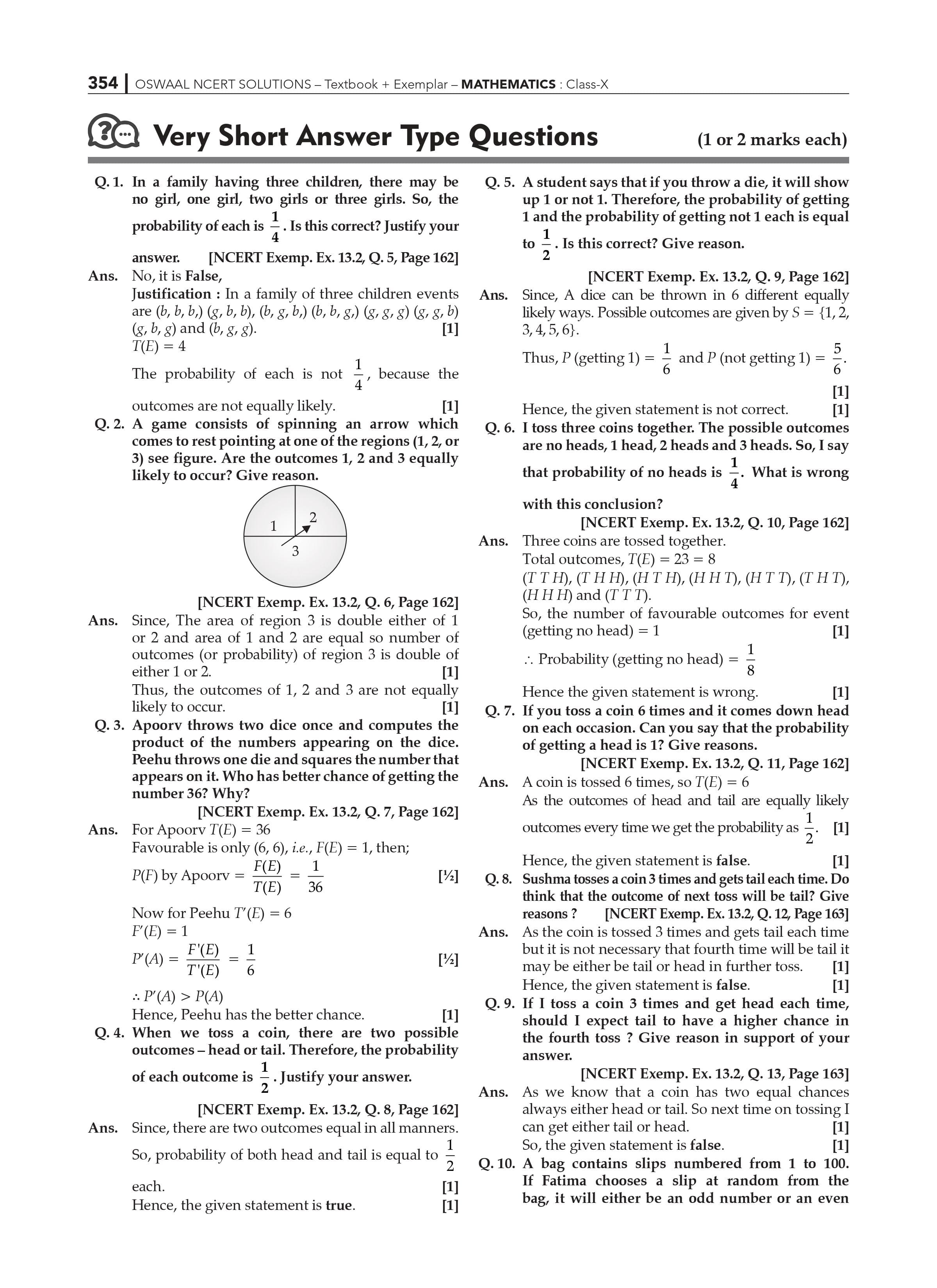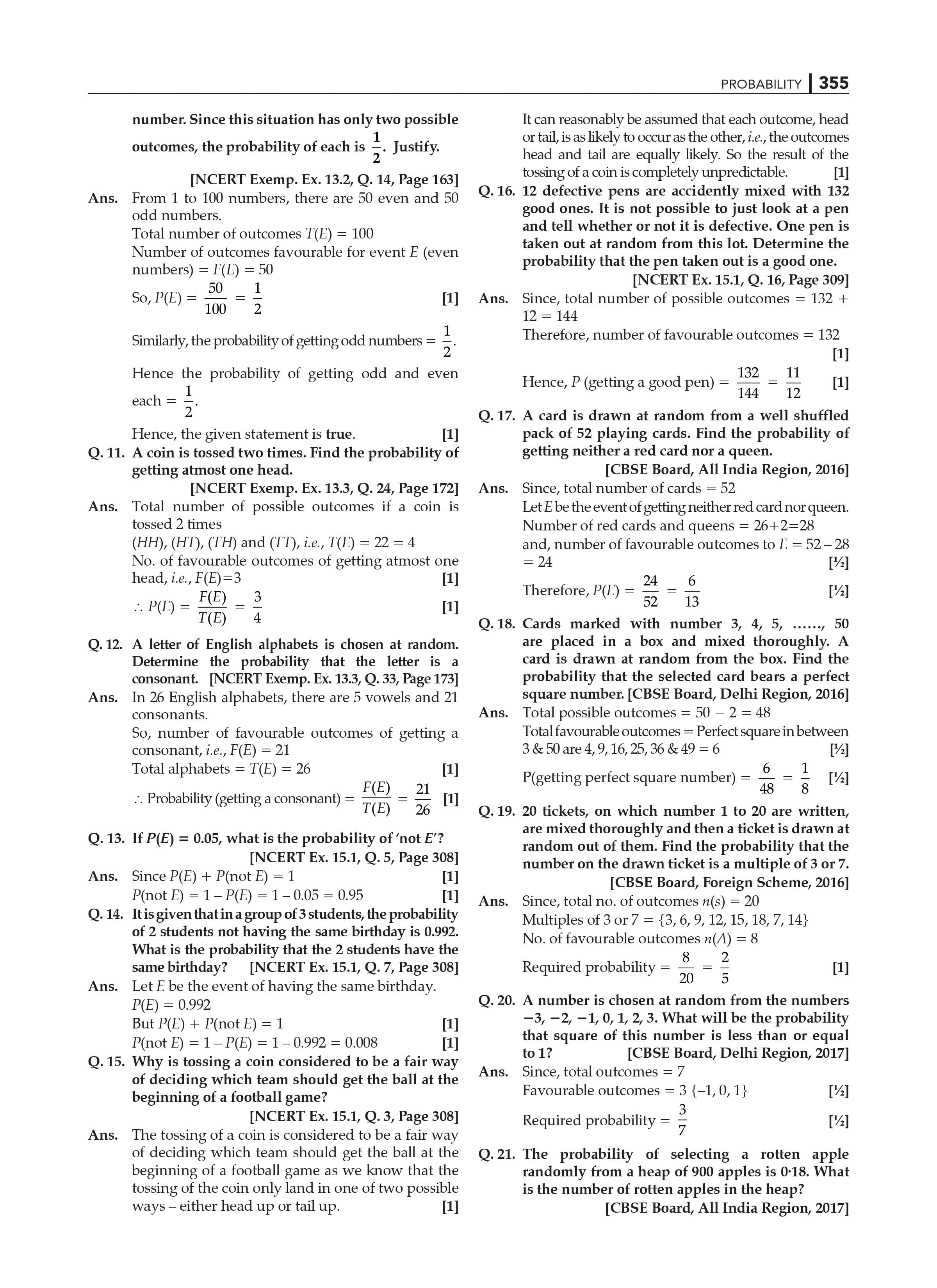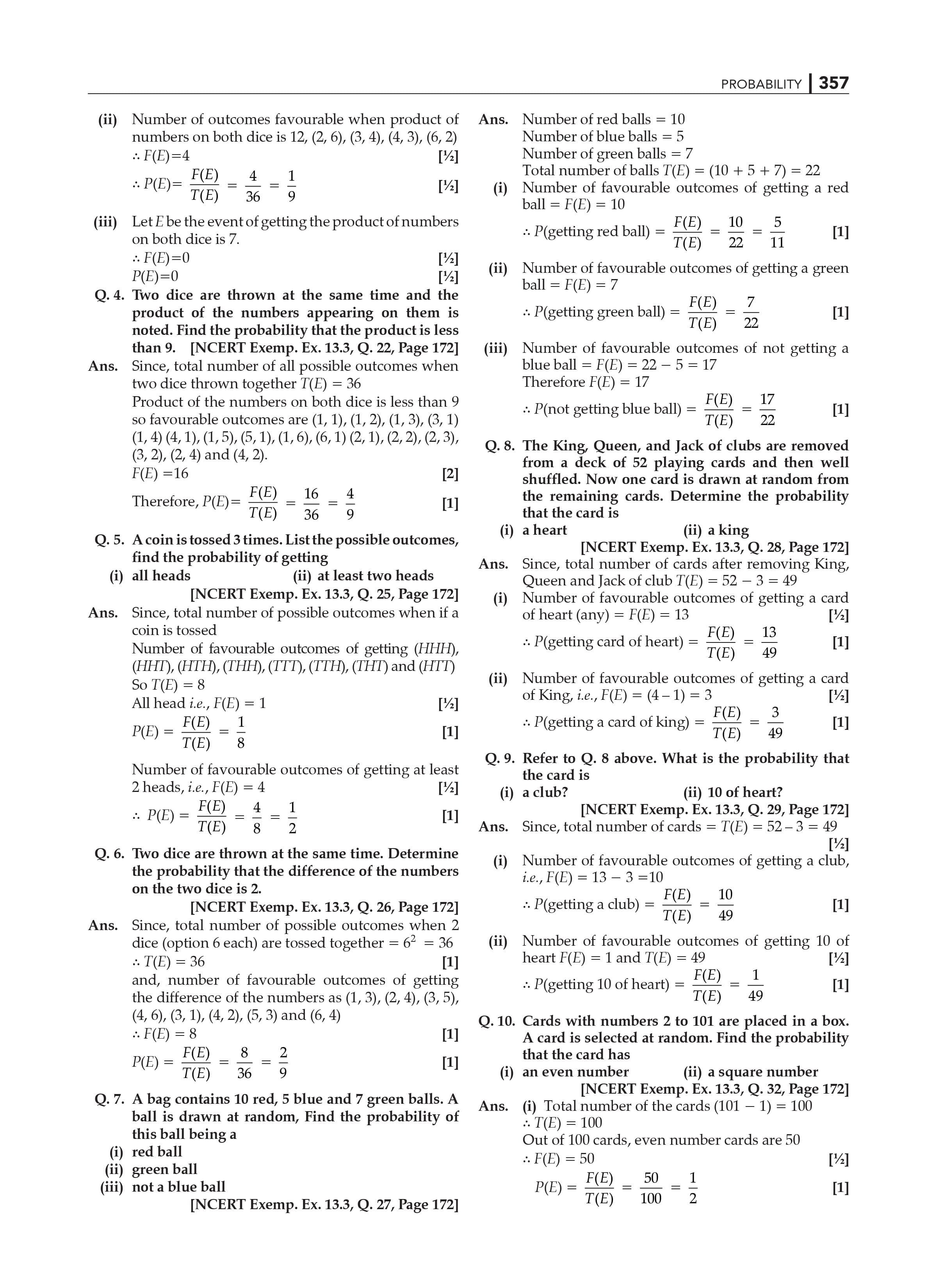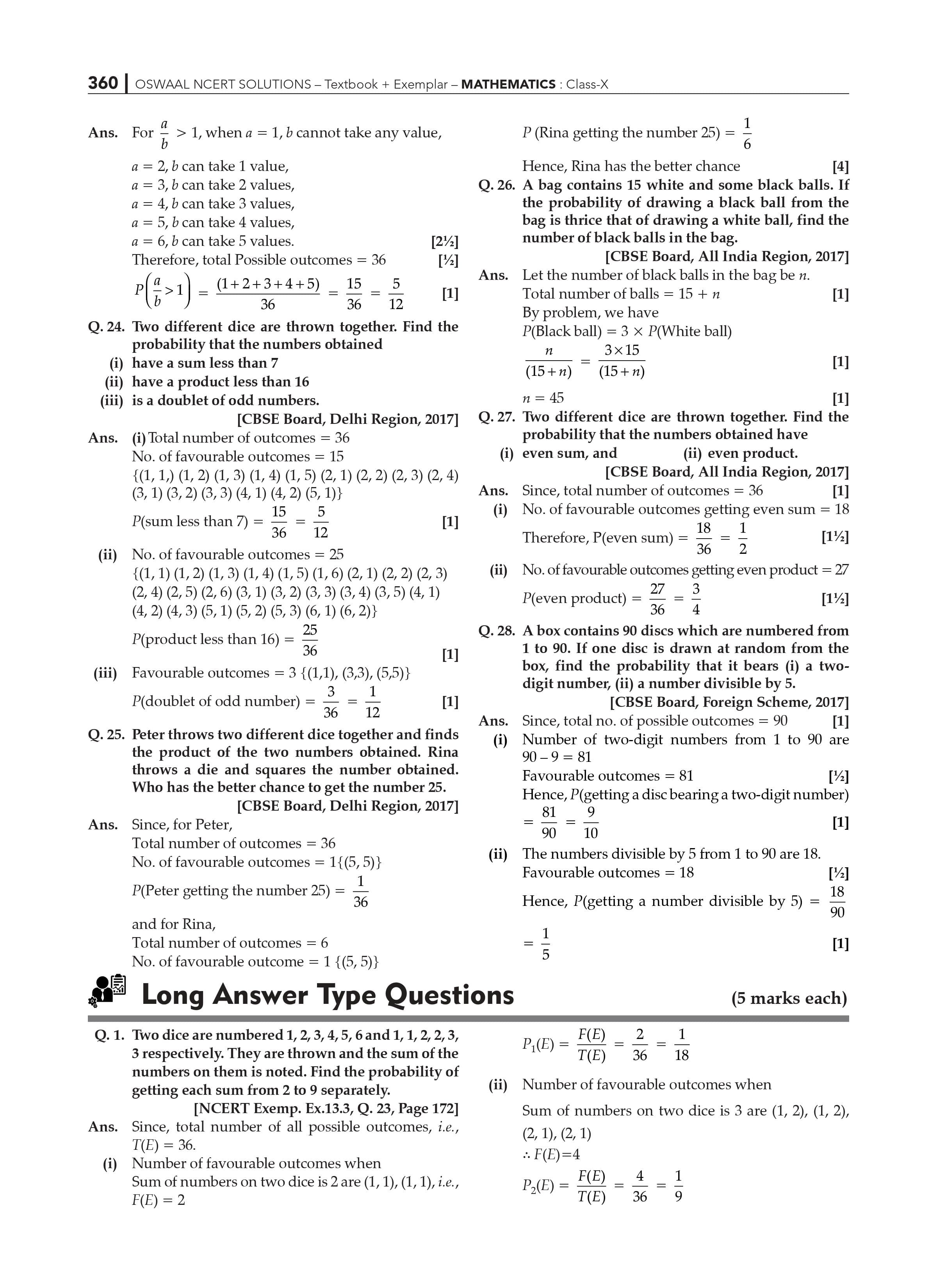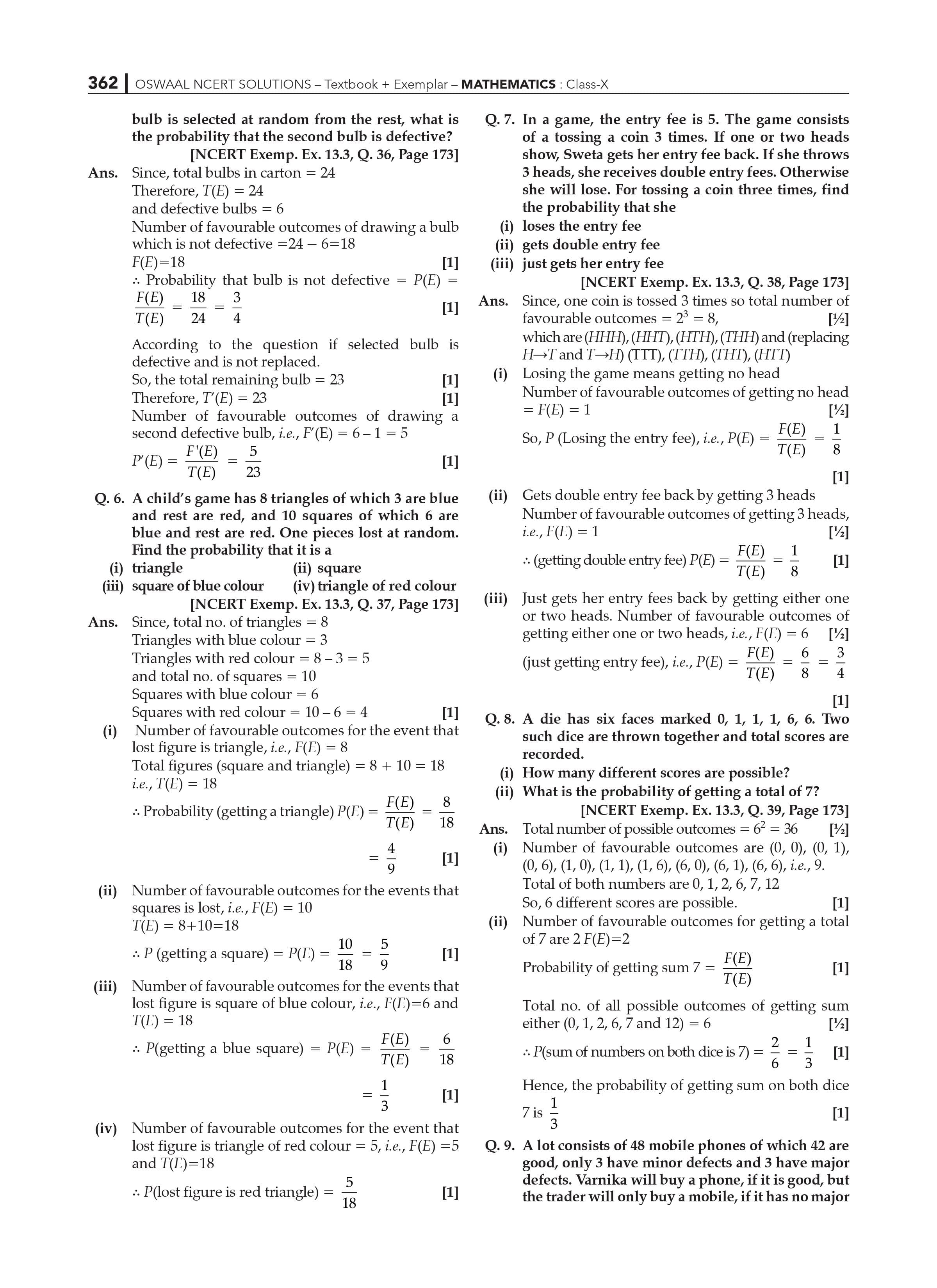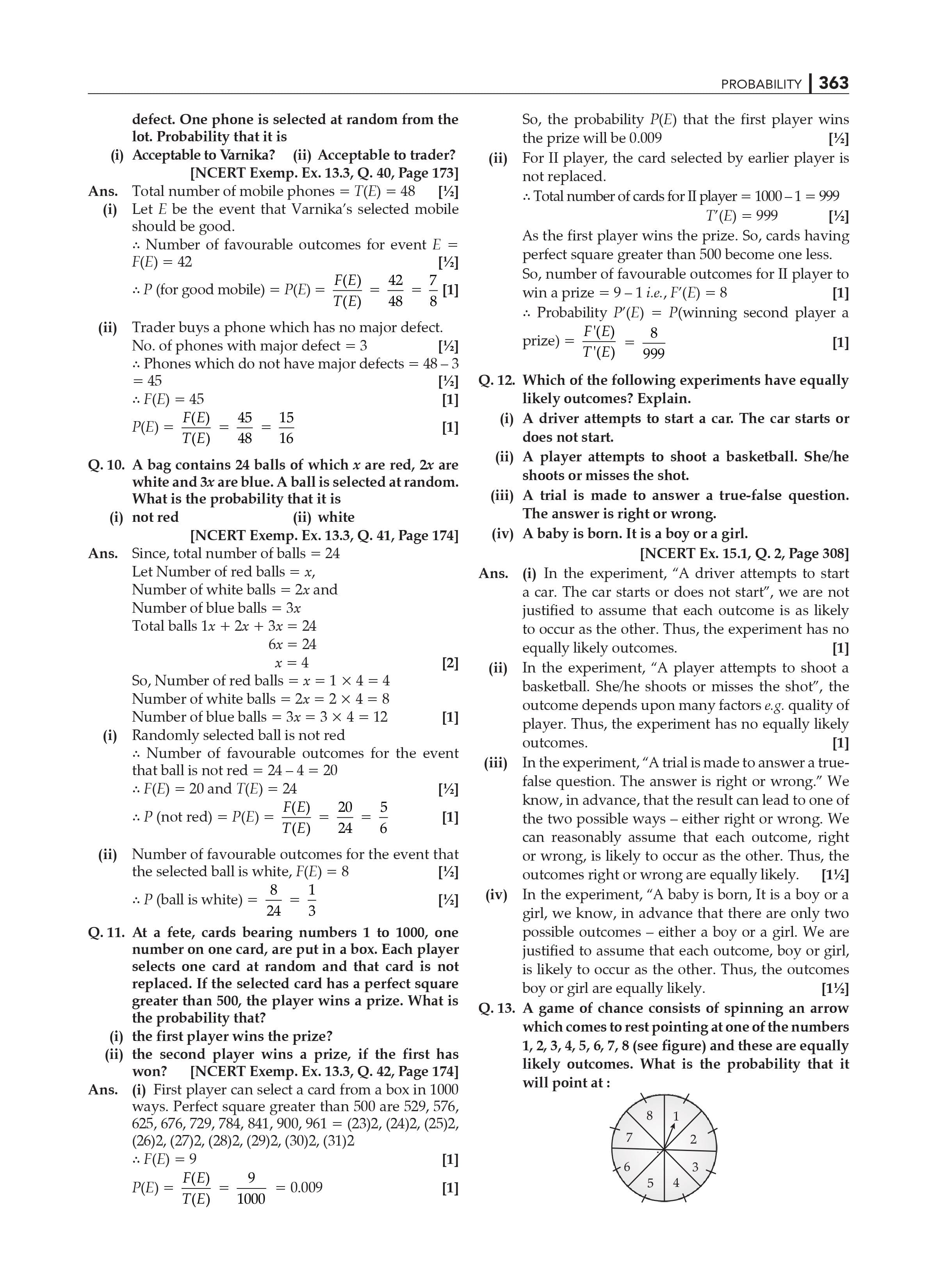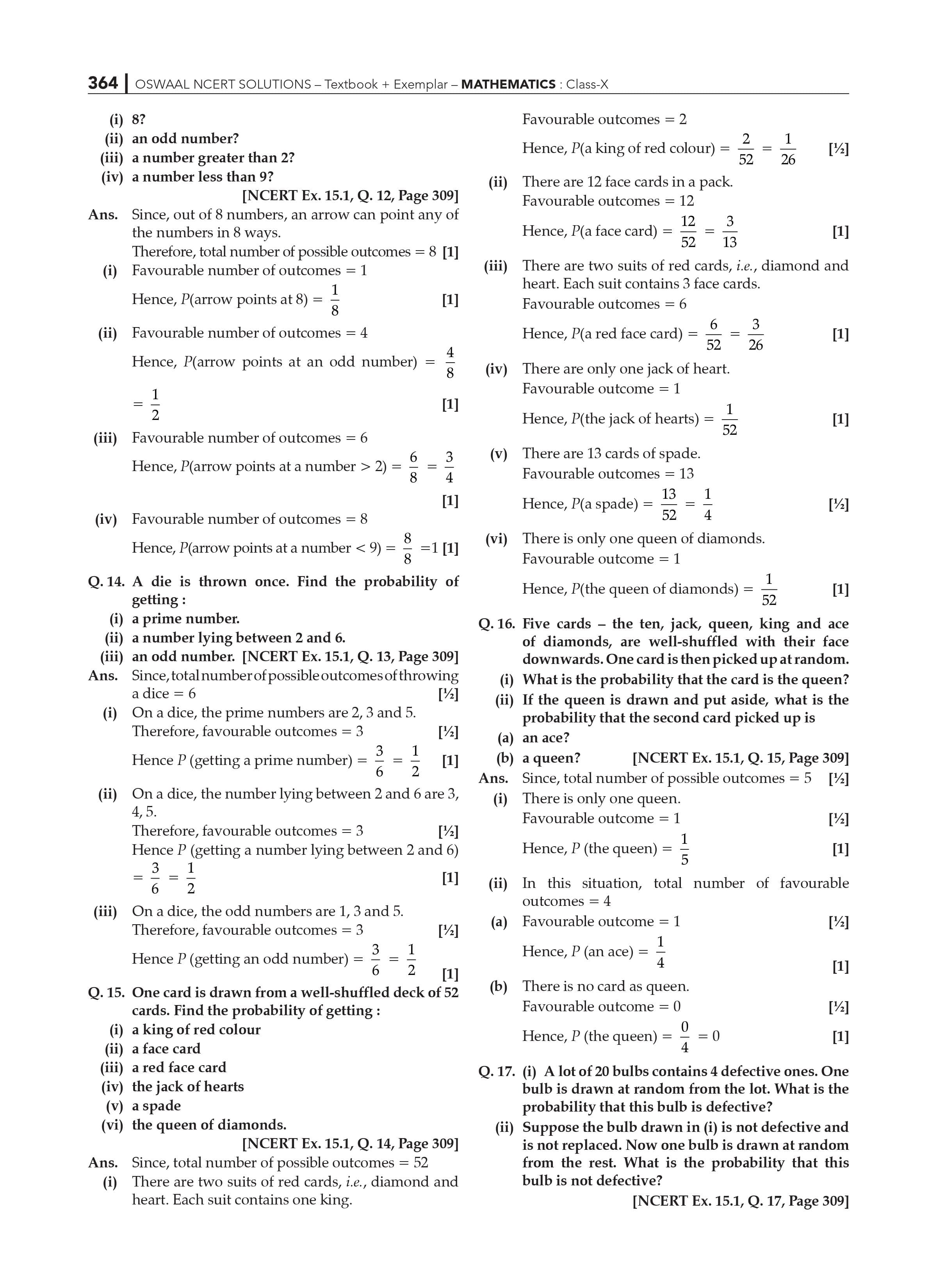Chapter15 Probability is a section in which the topic name is:

15 Probability.

15.1 An Overview

15.2 A Theoretical Approach

15.3 Conclusion

Introduction: Class 10 Maths Chapter 15

A probability is a number that expresses the chance or likelihood of an event occurring. Proportions ranging from 0 to 1 and percentages ranging from 0% to 100% can be used to express probabilities. An event with a probability of 0 has no chance of occurring, whereas an event with a probability of 1 is extremely certain to occur. A probability of 0.45 (45%) means that the event has a 45 percent chance of occurring. Probability is a discipline of mathematics concerned with numerical explanations of the likelihood of an occurrence or the veracity of a claim. In general, an event's probability is a number between 0 and 1, with 0 suggesting impossibility and 1 indicating certainty.

Probability is defined as the ratio of the number of favourable outcomes to the total number of possible outcomes of an event. The number of favorable outcomes can be expressed as in an experiment with 'n' outcomes, x is the number of possible outcomes. The formula for estimating the probability of an event is as follows:

P(E) = Favourable outcomes/total outcomes

(Theory Approach) History of Probability: The beginnings of probability theory can be traced back to games of chance and gambling. In 1654, a gambler's quarrel over the split of a stake between two players whose game was halted before it ended gave rise to probability. The subject was presented to notable mathematicians by a well-to-do gambler, the Chevalier de Mere, who shared his thoughts with Pierre de Fermat.

The RD Sharma Class 10 Textbook 2019 is based on the most recent CBSE CCE guidelines-required syllabus. The RD Sharma Class 10 pdf Maths book is intended for students enrolled in the Central Board of Secondary Education (CBSE) (CBSE). NCERT rules were followed in the development of RD Sharma's solutions. Our Maths experts have solved free RD Sharma Class 10 Solutions based on the (NCERT) CBSE norms. In Class 10 Math, students can simply improve their grades.

Real Life Examples of probability: Chance has anything to do with probability. It is the study of events that may or may not occur. Most of the time, we use it without even realising it. In everyday life, we don't solve genuine probability problems; instead, we utilise subjective probability to decide on a course of action or make a decision. Everything is a possibility, from weather forecasts to our chances of dying in an accident.

There is a step-by-step solution for each issue on this page. Students will gain an understanding of how to tackle problems by working on NCERT Solutions for Class 10. You may easily learn basic concepts better and faster with the assistance of these NCERT Solutions for Class 10 Maths. It is also a fantastic resource for supporting you in attaining good scores on the CBSE board exam.

Conclusion: Probability is a numerical estimate of the probability of an event occurring compared to a set of alternative events that do not. It is necessary to have a complete understanding of all possible outcomes.

Important questions for Class 10 Maths Chapter 15 Probability are provided here, weighted according to CBSE guidelines. The questions are based on the CBSE 2022-2023 Syllabus and the most recent exam pattern. Students studying for the CBSE class 10 board exams should go over these Probability questions to ensure that they receive full credit for the questions in this chapter.

 Extra Information: NCERT Exemplar for Class 10 Maths Chapter 15 CBSE Notes for Class 10 Maths Chapter 15

Frequently Asked Questions for CBSE Class 10 chapter 15- Probability:

1. Where can I find an exact NCERT Solution for Class 10 Maths Chapter 15 solution?

The correct solution can be found at Oswaal’s CBSE Books Class 10 for NCERT Solution for Class 10 Maths Chapter 15. Mathematicians have meticulously created the NCERT Textbook Solutions for Chapter 15. All of these solutions are based on the new CBSE term-by-term syllabus and rules, ensuring that students have a complete understanding of the material for their exams.

1. According to NCERT Solutions for Class 10 Maths Chapter 15, what does probability mean?

Probability, according to NCERT Solutions for Class 10 Maths Chapter 15, indicates the possibility of something happening. It's a branch of mathematics concerned with the likelihood of a random occurrence occurring. From 0 to 1, the value is expressed. Probability is a mathematical concept that predicts the likelihood of events happening.